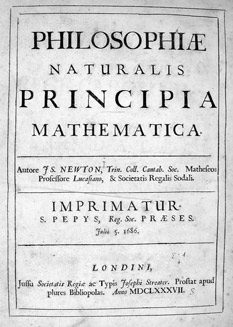# Principia : the mathematical principles of natural philosophy, by Isaac NewtonPhilosophiæ Naturalis Principia Mathematica, Latin for “Mathematical Principles of Natural Philosophy”, often referred to as simply the Principia, is a work in three books by Sir Isaac Newton, first published 5 July 1687. After annotating and correcting his personal copy of the first edition, Newton also published two further editions, in 1713 and 1726. The Principia states Newton’s laws of motion, forming the foundation of classical mechanics, also Newton’s law of universal gravitation, and a derivation of Kepler’s laws of planetary motion (which Kepler first obtained empirically). The Principia is “justly regarded as one of the most important works in the history of science”.

The French mathematical physicist Alexis Clairaut assessed it in 1747: “The famous book of mathematical Principles of natural Philosophy marked the epoch of a great revolution in physics. The method followed by its illustrious author Sir Newton … spread the light of mathematics on a science which up to then had remained in the darkness of conjectures and hypotheses.” A more recent assessment has been that while acceptance of Newton’s theories was not immediate, by the end of a century after publication in 1687, “no one could deny that” (out of the ‘Principia’) “a science had emerged that, at least in certain respects, so far exceeded anything that had ever gone before that it stood alone as the ultimate exemplar of science generally.”

In formulating his physical theories, Newton developed and used mathematical methods now included in the field of calculus. But the language of calculus as we know it was largely absent from the Principia; Newton gave many of his proofs in a geometric form of infinitesimal calculus, based on limits of ratios of vanishing small geometric quantities. In a revised conclusion to the Principia (see General Scholium), Newton used his expression that became famous, Hypotheses non fingo (“I contrive no hypotheses”). (Wikipedia)

Sari la bara de unelte